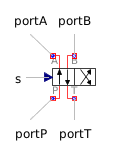Differential Pressure Sensor - MapleSim Help

Differential Pressure Sensor

Sense pressure drop between portsDescription The Differential Pressure Sensor component generates an output signal proportional to the pressure drop across the ports.Equations $y=\mathrm{toUnit}p$ $p={p}_{A}-{p}_{B}$ $\mathrm{mflow}={\mathrm{mflow}}_{A}=-{\mathrm{mflow}}_{B}=0\phantom{\rule[-0.0ex]{0.0em}{0.0ex}}\phantom{\rule[-0.0ex]{0.0em}{0.0ex}}$Variables

 Name Units Description Modelica ID $p$ $\mathrm{Pa}$ Pressure across component p $\mathrm{mflow}$ $\frac{\mathrm{kg}}{s}$ Mass flow rate through component mflow ${\mathrm{hflow}}_{X}$ $\frac{J}{\mathrm{kg}}$ Specific Enthalpy into port X hflowXConnections

 Name Description Modelica ID $\mathrm{portA}$ Upstream Pneumatic port portA $\mathrm{portB}$ Downstream Pneumatic port portB $y$ Real output; pressure in selected units yParameters

 Name Default Units Description Modelica ID $\mathrm{toUnit}$ $\mathrm{Pa}$ Selected pressure unit toUnit